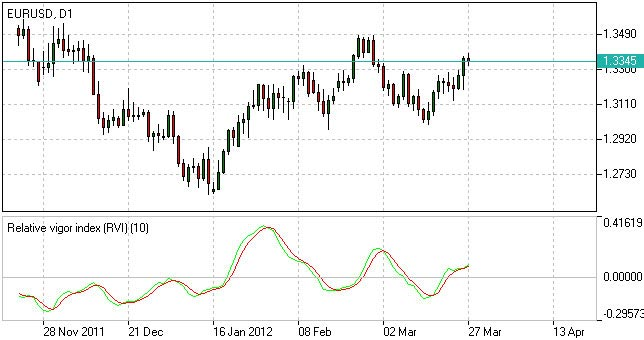Relative Vigor Index | RVI Indicator | Relative Vigor Index Formula | IFCM

# Relative Vigor Index - RVI Indicator

## What is Relative Vigor Index

Relative Vigor Index (RVI Indicator), developed by John Ehlers, is a technical indicator designed to determine price trend direction. The underlying logic is based on the assumption that close prices tend to be higher than open prices in a bullish environment and lower in a bearish environment.

which account to choose

## How to Use RVI Indicator

The Relative Vigor Index allows to identify the reinforcement of price changes (and therefore may be used within convergence/divergence patterns analysis):

• Generally the higher the indicator climbs, the stronger is the current relative price increase;
• Generally the lower the indicator falls, the stronger is the current relative price drop.

Together with its signal line (Red), a 4-period moving average of RVI, the indicator (Green) may help to identify changes in prevailing price developments:

• Crossing the signal line from above, the RVI signals a possible sell opportunity;
• Crossing the signal line from below, the RVI signals a possible buy opportunity.Relative Vigor Index - RVI Indicator

## Relative Vigor Index Formula (RVI Calculation)

The Relative Vigor Index indicator is calculated as the actual price change for a certain period divided by the maximum range of price changes in that period. To reduce the dependence on strong price fluctuations, the averaging was applied according to the algorithm of Simple Moving Average with the period of 10.

The Relative Vigor Index formula is as follows:

`Relative Vigor Index (1) = (Close - Open) / (High - Low)Relative Vigor Index (10) = 10-period SMA of Relative Vigor Index (1)`